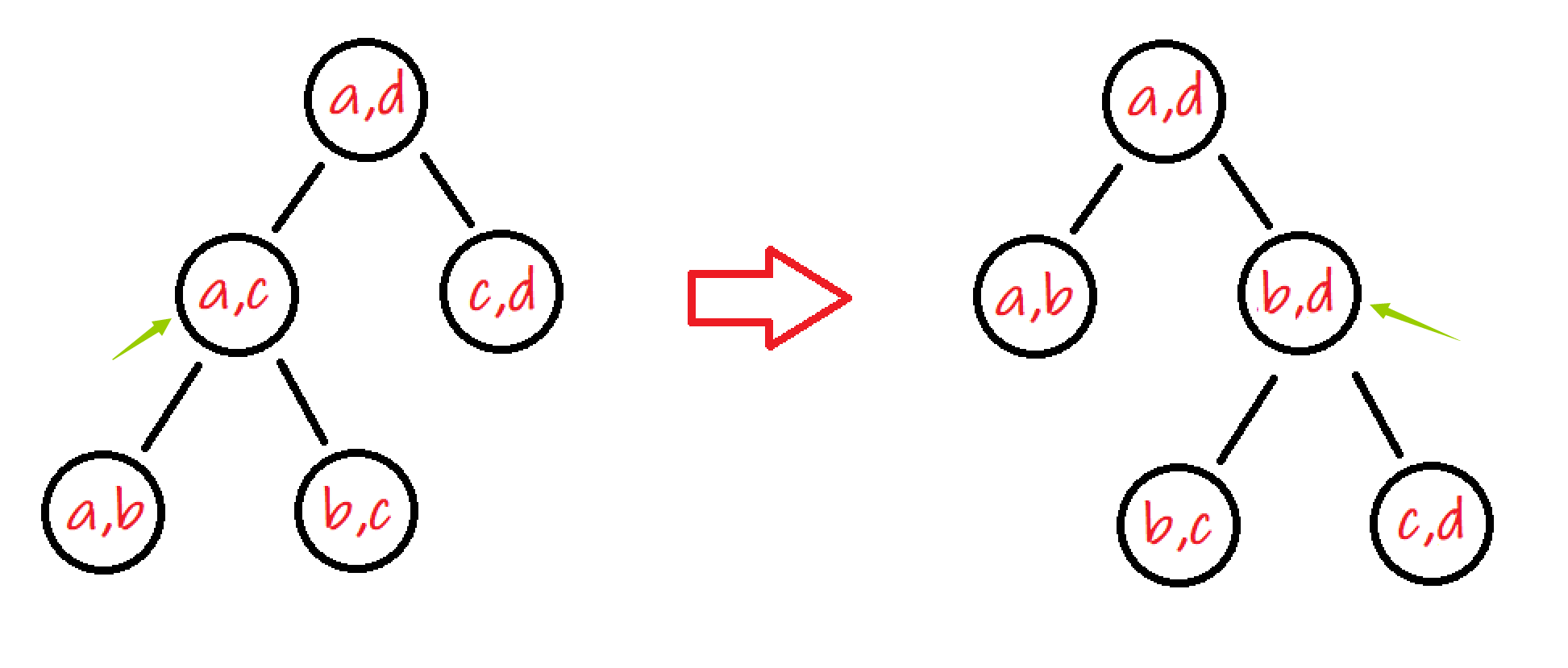$\texttt{HNOI2019}$ 终于改出来一道题目了…… 感谢 $JerryC$ 跟我一起讨论，不然我也看不懂题解。这题真的是 $\texttt{HNOI2019}$ 最可做的题啊，可想而知 $\texttt{HNOI2019}$ 有多么毒瘤了。(当然是我太蒟了)

### 3.$m>0$ 且只有第一问的情况

#### 一. 该节点在树中有父节点（左边的是原来的，右边的是经过了 $(a,c)$ 旋转的）

### Code:

#include <map>
#include <cmath>
#include <cstdio>
#include <vector>
#include <string>
#include <cstring>
#include <iostream>
#include <algorithm>
using namespace std;
typedef long long ll;

const int N=1e5+2;
const int MOD=1e9+7;

template <typename _Tp> inline void IN(_Tp&x) {
char ch;bool flag=0;x=0;
while(ch=getchar(),!isdigit(ch)) if(ch=='-') flag=1;
while(isdigit(ch)) x=x*10+ch-'0',ch=getchar();
if(flag) x=-x;
}

int W,n,Ans1,Ans2=1;
vector<int> G[N];
/*G[i] 表示与 i 相连接的点的集合*/
map<pair<int,int>,int> vis;
/*这个是为了方便快速找到代表 (a,c) 节点所用的 map*/

inline void solve() {//初始化
for(int i=2;i<n;++i)
G[i].push_back(i+1),G[i].push_back(i-1);
G.push_back(n),G.push_back(2);
G[n].push_back(n-1),G[n].push_back(1);
for(int i=1;i<=n;++i) sort(G[i].begin(),G[i].end());
}

int inv[N<<1],fct[N<<1],fci[N<<1];
/*分别对应逆元，阶乘，逆元的阶乘。主要用于计算组合数*/
inline int C(int n,int m) {
if(n<0||m<0||n<m) return 0;
return 1ll*fct[n]*fci[m]%MOD*fci[n-m]%MOD;
}
inline int Inv_C(int n,int m) {
if(n<0||m<0||n<m) return 0;
return 1ll*fci[n]*fct[m]%MOD*fct[n-m]%MOD;
}
inline int calc(int n,int m) {return C(n+m,n);}
inline int Inv_calc(int n,int m) {return Inv_C(n+m,n);}
/*上面的组合数不再赘述......*/

int root[N],fa[N<<1],sz[N<<1],ch[N<<1],tot;
/*root[i] 就是 S 集合中的区间 ai,ai+1 在树中的节点的编号*/
/*fa 表示父节点，sz 表示节点子树大小，ch 表示节点的左右儿子*/
void dfs(int&x,int f,int l,int r) {
if(r-l<=1) return;
x=++tot,sz[x]=1,fa[x]=f;
int p=lower_bound(G[r].begin(),G[r].end(),l+1)-G[r].begin();
/*找到这个 p*/
p=G[r][p],vis[make_pair(l,r)]=x;
/*找到 p 在原多边形中对应的点，并记录 l,r 在树中的点的编号*/
dfs(ch[x],x,l,p),dfs(ch[x],x,p,r);/*向下计算子树*/
sz[x]+=sz[ch[x]]+sz[ch[x]];/*统计子树大小*/
Ans2=1ll*Ans2*calc(sz[ch[x]],sz[ch[x]])%MOD;/*计算贡献*/
}

int main() {
IN(W),IN(n);
inv=inv=fct=fci=1;
for(int i=2;i<=n+n;++i) inv[i]=1ll*(MOD-MOD/i)*inv[MOD%i]%MOD;
for(int i=1;i<=n+n;++i) fct[i]=1ll*fct[i-1]*i%MOD;
for(int i=1;i<=n+n;++i) fci[i]=1ll*fci[i-1]*inv[i]%MOD;
/*以上为初始化逆元，阶乘，逆元的阶乘*/
for(int i=1;i<=n-3;++i) {
int x,y;IN(x),IN(y);
G[x].push_back(y),G[y].push_back(x);
}
solve(),Ans1=n-1-G[n].size();
for(int i=0,len=G[n].size();i<len-1;++i)
dfs(root[i],0,G[n][i],G[n][i+1]);/*计算每个区间 ai,ai+1 的树*/
int Size=0;
for(int i=0,len=G[n].size();i<len-1;++i)
Ans2=1ll*Ans2*calc(Size,sz[root[i]])%MOD,Size+=sz[root[i]];
/*统计答案*/
if(!W) printf("%d\n",Ans1);
else printf("%d %d\n",Ans1,Ans2);
int q;IN(q);
while(q--) {
int a,b;IN(a),IN(b);
if(a>b) a^=b^=a^=b;
int x=vis[make_pair(a,b)];/*找到在原树中 a,b 所代表的节点*/
if(!W) {printf("%d\n",Ans1-(fa[x]?0:1));continue;}
else {
int nowans1=Ans2;
if(fa[x]) {
int y=fa[x],k=ch[y]==x;
nowans1=1ll*nowans1*Inv_calc(sz[ch[x]],sz[ch[x]])%MOD;
nowans1=1ll*nowans1*Inv_calc(sz[ch[y]],sz[ch[y]])%MOD;
nowans1=1ll*nowans1*calc(sz[ch[x][!k]],sz[ch[y][!k]])%MOD;
nowans1=1ll*nowans1*calc(1+sz[ch[y][!k]]+sz[ch[x][!k]],sz[ch[x][k]])%MOD;
/*除掉贡献与增加贡献*/
} else {
nowans1=1ll*nowans1*Inv_calc(sz[ch[x]],sz[ch[x]])%MOD;
nowans1=1ll*nowans1*Inv_calc(Size-sz[x],sz[x])%MOD;
nowans1=1ll*nowans1*calc(Size-sz[x],sz[ch[x]])%MOD;
nowans1=1ll*nowans1*calc(Size-sz[x]+sz[ch[x]],sz[ch[x]])%MOD;
/*除掉贡献与增加贡献*/
}
printf("%d %d\n",Ans1-(fa[x]?0:1),nowans1);/*输出答案*/
}
}
return 0;
}


QAQ